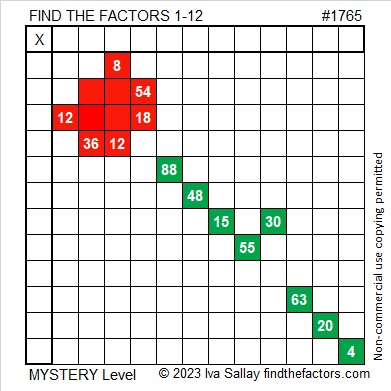# 1765 On This Memorial Day

### Today’s Puzzle:

This weekend I laid a bouquet of red and white flowers on my husband’s grave and decided to make a red rose Memorial Day puzzle for the blog as well. It is a mystery-level puzzle.

Write the number from 1 to 12 in both the first column and the top row so that those numbers are the factors of the given clues. There is only one solution.### Factors of 1765:

• 1765 is a composite number.
• Prime factorization: 1765 = 5 × 353.
• 1765 has no exponents greater than 1 in its prime factorization, so √1765 cannot be simplified.
• The exponents in the prime factorization are 1 and 1. Adding one to each exponent and multiplying we get (1 + 1)(1 + 1) = 2 × 2 = 4. Therefore 1765 has exactly 4 factors.
• The factors of 1765 are outlined with their factor pair partners in the graphic below.### More About the Number 1765:

1765 is the sum of two squares in two different ways:
42² + 1² = 1765, and
33² + 26² = 1765.

1765 is the hypotenuse of FOUR Pythagorean triples:
84 1763 1765, calculated from 2(42)(1), 42² – 1², 42² + 1²,
413 1716 1765, calculated from 33² – 26², 2(33)(26), 33² + 26²,
1059-1412-1765, which is (3-4-5) times 353, and
1125-1360-1765, which is 5 times (225-272-353).

1765 is a digitally powerful number:
1⁴ + 7³ + 6⁴ + 5³ = 1765.

1765 is a palindrome in a couple of different bases:
It’s A5A base 13 because 10(13²) + 5(13) + 10(1) = 1765, and
it’s 1D1 base 36 because 1(36²) + 13(36) + 1(1) = 1765.